Technical Article

# Don’t Get Lost in Deep Space: Understanding Quaternions

March 10, 2017 by Mark Hughes

## Quaternions are mathematical operators that are used to rotate and stretch vectors. This article provides an overview to aid in understanding the need for quaternions.

Quaternions are mathematical operators that are used to rotate and stretch vectors. This article provides an overview to aid in understanding the need for quaternions in applications like space navigation.

Accurately locating, shifting, and rotating objects in space can be done in a variety of ways. The more familiar and easy to visualize roll, pitch, and yaw are limited and should be replaced in certain cases with the more robust quaternion. As the position and orientation of the object change, a mathematical device known as a quaternion is used to rotate and scale the original vector.

Objects in a three-dimensional space can be located in a coordinate system with three numbers that extend from the origin of the coordinate system to a point in space, creating a position $$\textbf{r}=(x,y,z)$$ vector. If the position of the object changes, the vector will be in a new location and perhaps be of a new length. We need a way to measure or calculate the changes between two vectors.

### The Problem with Roll, Pitch, and Yaw

Most readers are likely aware that objects that rotate through space can be described by their angle of rotation along three axes. Any rotation in space can be described by a combination of these rotations.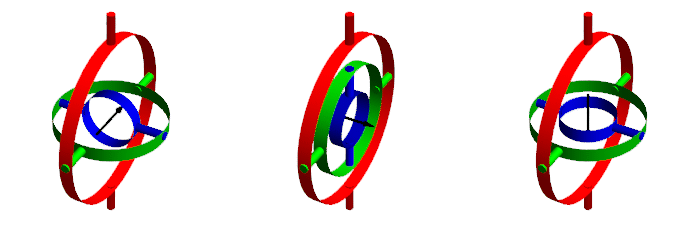##### Gimbals above provide yaw, roll, and pitch movements. Image credit: Mark Hughes (made with Mathematica)

The rotation axes are not always independent, and solutions are not always unique. It is possible for the plane of two gimbals to align, and a condition known as gimbal lock occurs. In gimbal lock, two of three gimbals are parallel or very nearly parallel, and what began as three degrees of freedom (yaw, pitch, and roll) reduces to two degrees of freedom—two axes of rotation can describe the same rotational motion. At the same time, one degree of freedom is lost and that information disappears. Once gimbal lock occurs, it is impossible to reorient the axes without an external reference.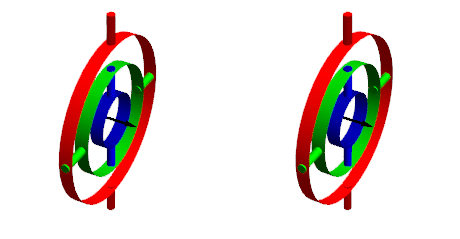##### When the green circle is aligned or close to aligned with the red circle, movements of the red circle and the blue circle coincide and "gimbal-lock" occurs. Image credit: Mark Hughes.

You might remember hearing the term gimbal lock from the movie about the Apollo 13 mission. Had gimbal lock occurred after the explosion, the astronauts' inertial measurement unit would have lost track of their position in the celestial sphere, negatively affecting their already desperate situation.

Now for a look at the mathematics behind gimbal lock.

***Note to readers: To maintain readability on small devices, all instances of cos(x) have been replaced with Cx, and all instances of sin(x) replaced with Sx. You can view the full equations by clicking on the images.

Rotating a vector $$\textbf{r}=(x, y, z)$$ around a single axis requires a rotation matrix. The three-axis gimbal shown above has three axes that correspond to three rotation matrices.

##### Shown above: x-axis rotation around the angle γ, a y-axis rotation around the angle β, and a z-axis rotation around the angle α.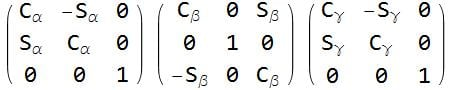##### Shown above are three separate 3×3 transformation matrices. (A z-y-z transformation around angles α, β, γ)

Mathematically, a 3×3 rotation matrix is the product of three sequential rotations.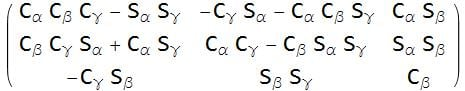##### 3×3 matrices, when consecutively multiplied, result in a single 3×3 matrix. Shown above is a z-y-z rotation around angles α, β, γ

Multiple transformation matrices exist, and they can be applied in various orders. The twelve rotation sequences can be divided into two categories: Proper Euler angles, where one axis of rotation is repeated (x-z-x, x-y-x, y-x-y, y-z-y, z-y-z, z-x-z), and Tait-Bryan angles, which rotate around all axes (x-z-y, x-y-z, y-x-z, y-z-x, z-y-x, z-x-y).

I arbitrarily chose the z-y-x transformation matrix to work with in the examples below.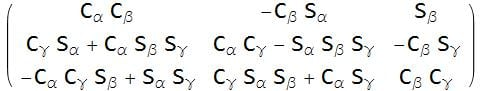##### Above is a transformation matrix that rotates z-y-x around α, β, γ

Gimbal lock becomes mathematically apparent in our example matrix when $$\beta=\frac{\pi}{2}$$ is substituted into a z-y-x transformation matrix (other transformation matrices fail with different conditions). As the angle $$\beta \to \frac{\pi}{2}$$, $$Cos(\beta) \to 0$$ and $$Sin(\beta) \to 1$$. You can see below the simplifying effect that has on the matrix.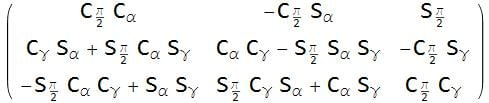##### As β is evaluated at or near $$\frac{\pi}{2}$$, the $$Cos(\frac{\pi}{2})$$ causes several items in the matrix to go to zero.

In the example above, substituting $$\beta=\frac{\pi}{2}$$ and $$Cos(\frac{\pi}{2})=1$$ into the original matrix eliminates several terms.

Another way to look at the problem is to take the original transformation matrix (I again chose z-y-x) and use trigonometric identities to bring variables together inside the trigonometric function.  Pay attention to the initial interdependence of the angles.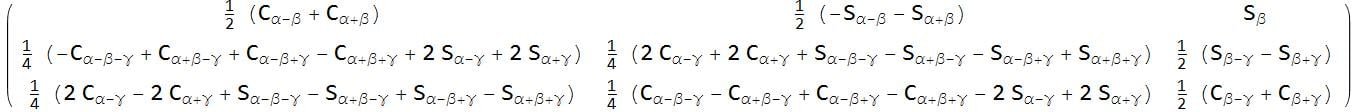##### z-y-x roll-pitch-yaw transformation matrix with trigonometric identities applied to show the interdependence of the variables α, β, γ

After substitution, the only relationship between angles that survives is $$\alpha+\gamma$$.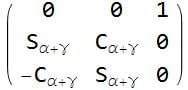##### The only variable that can now change is $$\alpha+\gamma$$ (with $$\beta=\frac{\pi}{2}$$).

By fixing $$\beta=\frac{\pi}{2}$$ we have eliminated all cases of $$\alpha-\gamma$$, sacrificing a degree of freedom.

While it is easy to visualize roll, pitch, and yaw, if you are designing a system that is able to freely point in any direction in space, you will eventually encounter gimbal lock.

### Enter Quaternions

William Hamilton invented quaternions in 1843 as a method to allow him to multiply and divide vectors, rotating and stretching them.

What I present below is intended to be illustrative, but by no means mathematically rigorous. It should be sufficient to allow you to understand quaternions at an introductory level for a computer science and engineering setting. It is not meant to be sufficient for a mathematics class. If you require more in-depth information, the following books on the topic were recommended by California State University, Fullerton, Professor of Physics and Mathematics, Dr. Alfonso Agnew:

Hamilton's discovery was that while there was no obvious way to multiply and divide two sets of three numbers that resulted in three numbers (vectors that might represent a coordinate), it was possible to multiply and divide two sets of four numbers and be left with four numbers. A quaternion became the quotient of two sets of four numbers and consists of one scalar number and one vector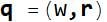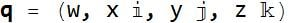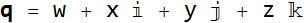Where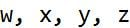are real numbers, and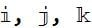are quaternion units.

The direction between any two points can be expressed by three numbers that individually lie in the range (-1,1) and whose collective magnitude is $$(-1\leq x\leq 1, -1 \leq y \leq 1, -1 \leq z \leq 1)$$ and $$\sqrt{x^2+y^2+z^2}=1$$. Together, those four numbers create a quaternion that describes rotation and distance.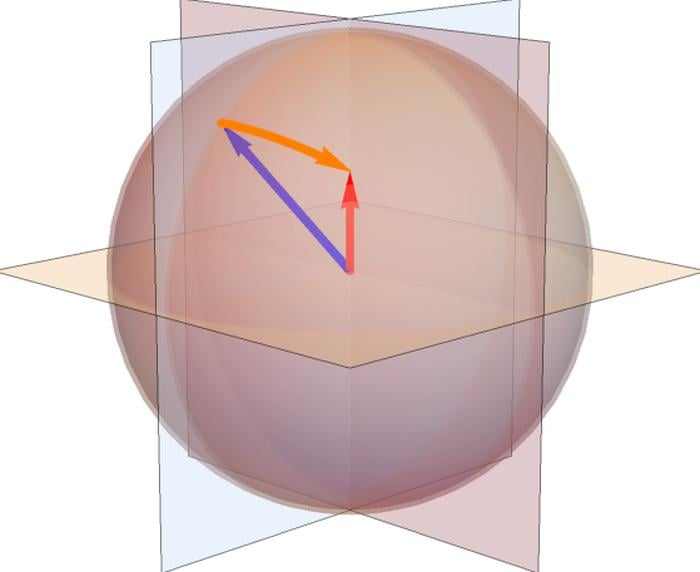##### In this image, the orange quaternion is applied to the blue position vector and results in the red position vector.

Quaternions provide the information necessary to rotate a vector with just four numbers instead of the nine needed with a rotation matrix.

If you are comfortable with math and matrix notation, make the leap to quaternion math below and skip the review of complex numbers and matrix mathematics in the next two sections.

### Complex Numbers

See AAC's textbook Volume 2 — Chapter 2.

Complex numbers were invented to allow solutions to problems that have no real number solution. Problems of the sort $$x^2=-1$$ had no solution until $$\sqrt{-1}$$ was invented.  Complex numbers can be imagined to lie on a plane, with the real part of the number expressed along the horizontal axis and the imaginary part of the number expressed along the vertical axis.  In Cartesian coordinates, they are typically expressed in a form similar to $$x+yi$$, or $$(x, y)$$.  Two complex numbers can be added, subtracted, multiplied, and divided.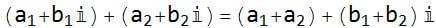#### Subtraction: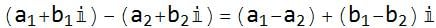#### Multiplication: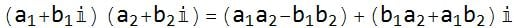#### Division: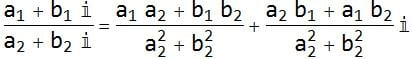#### Rotation (without scaling):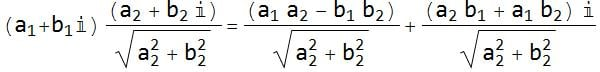An example clockwise $$\frac{\pi}{2}$$ (90°) rotation of $$2+3i$$ is: $$(2+3i)(0+i)=(2\times0)+(2\times i)+(3i\times0)+(3i\times i)=(3(\sqrt{-1})^2+2i)=(-3+2i)$$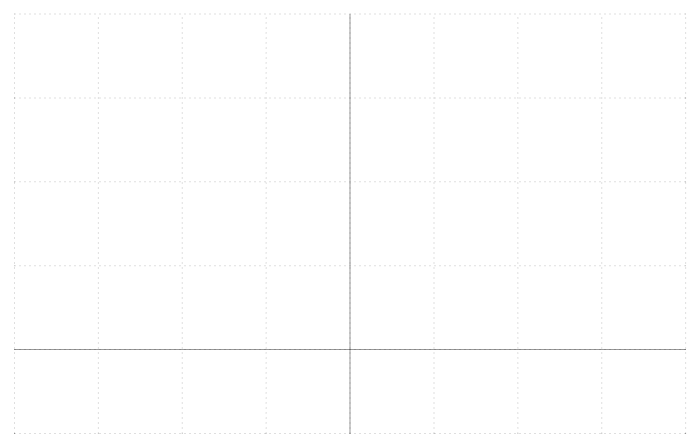The complex number 2+3i is rotated $$\frac{\pi}{2}$$ (90°) by multiplying by 0+1i.

Euler developed a method for rotating complex numbers in the complex polar plane that Hamilton built his ideas upon.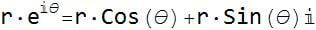While this is far from a full treatment on the subject of complex numbers, it provides a stepping stone towards quaternions in the following ways:

• Complex numbers can be added, subtracted, multiplied, and divided fairly easily and without the use of trigonometry (although the complex numbers can be expressed in polar form or decomposed from polar form into real and imaginary parts with cos and sin respectively).
• Multiplying a complex number by the imaginary unit "i" produces a quarter-revolution.  Similarly with quaternions, multiplying any two quaternion units together will result in a revolution around an axis that is perpendicular to the two initial axes.

### Vocabulary and Matrix Mathematics

Scalar

A scalar is a number that represents the position along a common scale or axis. Scalar variables have no special formatting applied to them.

Vector

A vector is a list of ordered numbers that describe position along a scale in a particular direction.  It is visualized as a straight line with length and direction. Vector variables are shown in bold $$\textbf{r}$$ throughout this article and occasionally with overscript arrows elsewhere on the site ($$\vec{r}$$) and in certain texts.  Vectors can have two or more elements.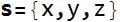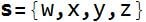Multiple vectors are distinguished by different variable names, or bysubscripts.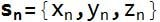or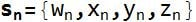Cross Product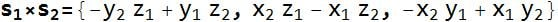Dot Product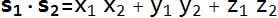Length

The length of a vector is the straight line distance from its start to its end. Mathematically it is given as the square root of the sum of the square of the individual elements.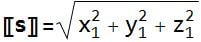Matrix

A matrix is an array of individual elements that can be multiplied by a vector to transform it.  Matrices can translate, rotate, and scale vectors.  Shown below is a generic 3×3 matrix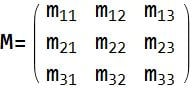Matrix M applied to vector x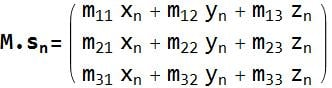Rotation Matrices

Rotation matrices can change the direction that vectors point, reorienting them in space.  The following matrices rotate vectors around the cartesian axes without scaling them.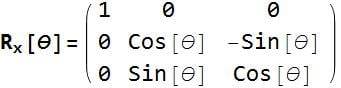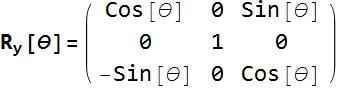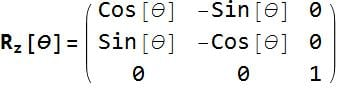Matrix A multiplied by Matrix B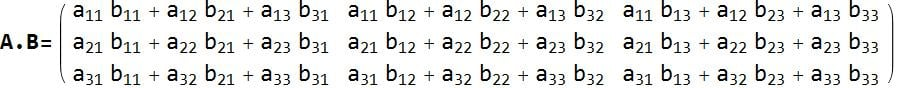This section is a brief review of vector and matrix mathematics and was provided as a contrast to the Quaternion rules below.

### The Leap to Quaternions

As mentioned earlier, quaternions are composed of a scalar and a vector.Since both scalars and vectors are present in a quaternion, the mathematical rules used to work with them are a combination of scalar and vector mathematics.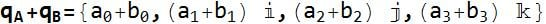(Noncommutative) Quaternion Multiplication

The result of multiplying two quaternions is a new quaternion.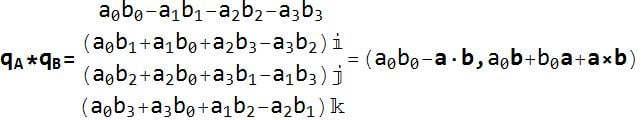Quaternion Inner Product

The quaternion inner product is a scalar that is found by multiplying corresponding real coefficients of two quaternions.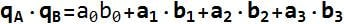Quaternion Conjugate

Each quaternion has an opposite that is found by negating the coefficients of the vector part of the quaternion only.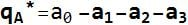Quaternion Norm

A quaternion should typically always lie along the unit sphere.  The norm should equal 1.  If your quaternion is drifting away from the unit sphere, you can divide each element of the quaternion by the norm to return to the unit sphere.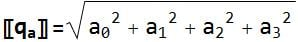Quaternion to Rotation Matrix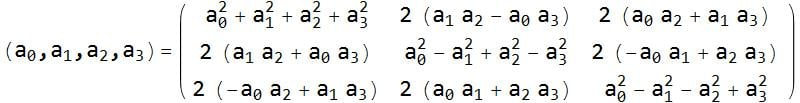More on the History of Quaternions

### Conclusion

Quaternions are an alternate way to describe orientation or rotations in 3D space using an ordered set of four numbers. They have the ability to uniquely describe any three-dimensional rotation about an arbitrary axis and do not suffer from gimbal lock. If you have an application where a sensor or object is capable of movement anywhere in 3D space, they are superior to Euler angles for tracking objects.•nsaspook March 10, 2017
•Sarvio Valente May 24, 2017
•Mark Hughes May 24, 2017